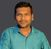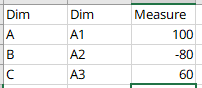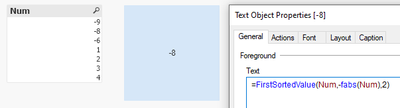# App Development

Announcements
Qlik Cloud Maintenance is scheduled between March 27-30. Visit Qlik Cloud Status page for more details.
cancel
Showing results for
Did you mean:Contributor

## How to find the second absolute value with its sign

Hi,

I am trying to find the second maxium absolut value with respect to its dimension. So far I have managed to find its absolute value with below expression but I need to know what is its sign (positive (+) or negative (-)).

Does any one have an idea?

Max(Aggr(fAbs(Measure), firstDimension, secondDimension), 2)

Labels (5)

• ### set Analysis Measures

1 Solution

Accepted SolutionsMVP

may be you have to use Aggr if you want it based on dimensions

=FirstSortedValue(aggr(sum(Measure),Dim1,Dim1),-fabs(aggr(sum(Measure),Dim1,Dim1)),2)

Where Measure,Dim1 & Dim2 are Your actual measure and dimensions respectively

8 RepliesSpecialist

Hi

you can try like

Num(Max(Aggr(Measure, firstDimension, secondDimension), 2),  '#,##0;(#,##0)')

if number is negative(-) then it is show under  bracket like (1235)

if number is positive (+) then it is show without bracket like 1234.Contributor
Author

Hi,

Your suggested expression will alwyas give me the second maximum value. I am trying to find the second Maximum absolute value. If I have a table like below, I would like to find -80. If I use your expression I will get 60.MVP

Try using FirstSortedValue(), like:

=FirstSortedValue(Num,-fabs(Num),2)Contributor
Author

I dont think your expression would work. You can try it in this dummy table where -80 should be the output.MVP

How exactly you are trying to get the output, in KPI object or in the same chart as a new column? Could you post your expected output in picture?Contributor
Author

I would like to show it as single value for kpi

In the screenshot that you attached, i don't see any dimension to aggregate on. I need to show the second maximum absolute value with respect to two dimension column.MVP

may be you have to use Aggr if you want it based on dimensions

=FirstSortedValue(aggr(sum(Measure),Dim1,Dim1),-fabs(aggr(sum(Measure),Dim1,Dim1)),2)

Where Measure,Dim1 & Dim2 are Your actual measure and dimensions respectivelyContributor
Author

Perfect. This one worked 🙂Tags
Community Browser